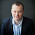## Introduction

Sometimes, there are workbooks where you don't have a granularity on a daily basis available but on a higher level. For, instance on a weekly or a monthly level. The problem is that the normal time intelligence function only works on a date dimension on a day granularity) and not a higher level of granularity. In this blog post I'll write down some of the time intelligence based on a monthly basis period dimension. The key of this dimension is based on the format YYYYMM.

## Netrevenue

First thing is always create an implicit summary of the measure, in this case the Net revenue. This is a best practice and has many advantages like disconnecting the busnesslogic from the business layer. For more best practices I'll advise you to take a look at this blogpost.

```       ```
SumNR:=SUM(FactSalesPerMonth[NetRevenue])
```
```

## QuarterToDate (QTD)

One requirement that is often heard is that customers want a QuarterToDate functionality. So in case of month January, February and March the values do add up and it is reset when April starts.

```       ```
```SumNetRevenueQTD:=CALCULATE ([SumNR];
FILTER(ALL(DimPeriodMonth);
DimPeriodMonth[CalendarYear] = VALUES(DimPeriodMonth[CalendarYear]) &&
DimPeriodMonth[Quarter] = VALUES(DimPeriodMonth[Quarter]) &&
DimPeriodMonth[MonthNumber] <= MAX(DimPeriodMonth[MonthNumber])
)
)
```

## YearToDate (YTD)

Yet another one is the YearToDate calculation. This calculation is used to summarize the numbers in a year until a certain point in time.

```       ```
SumNRYTD:=CALCULATE ([SumNR];
FILTER(ALL(DimPeriodMonth);
DimPeriodMonth[CalendarYear] = VALUES(DimPeriodMonth[CalendarYear]) &&
DimPeriodMonth[MonthNumber] <= MAX(DimPeriodMonth[MonthNumber])
)
)
```
```

## Last Year (LY)

sometimes, customers wants to compare the numbers with last year. This can be done with the following expression:

```       ```
SumNRLY:=CALCULATE([SumNR];
FILTER(ALL(DimPeriodMonth);
DimPeriodMonth[MonthNumber] = MAX(DimPeriodMonth[MonthNumber]) &&
DimPeriodMonth[CalendarYear] = MAX(DimPeriodMonth[CalendarYear]) - 1
)
)       ```
```

## QuarterToDate LastYear

The following DAX expression can be used to calculate the Quarter To date, not for this year, but for the year before the this year. And 'this year' depends of the context of the calculation, off course.

```       ```
SumNRQTDLY:=CALCULATE ([SumNR];
FILTER(ALL(DimPeriodMonth);
DimPeriodMonth[CalendarYear] = VALUES(DimPeriodMonth[CalendarYear]) - 1 &&
DimPeriodMonth[Quarter] = VALUES(DimPeriodMonth[Quarter]) &&
DimPeriodMonth[MonthNumber] <= MAX(DimPeriodMonth[MonthNumber])
)
)
```
```

## YearToDate LastYear

And the last one I would like to mention is the Year To date but for the last year. This DAX expression calculates the sum of last year until a certain point in time (depending on the context of the calculation)

```       ```
SumNRYTDLY:=CALCULATE ([SumNR];
FILTER(ALL(DimPeriodMonth);
DimPeriodMonth[CalendarYear] = VALUES(DimPeriodMonth[CalendarYear])-1 &&
DimPeriodMonth[MonthNumber] <= MAX(DimPeriodMonth[MonthNumber])
)
)
```
```

## Conclusion

This are some handy DAX expression that you can use in your calculations.

Greetz,
Hennie

#### 2 opmerkingen:

1.Hi,
Nice post I have read ever. I have a query. Could you please send me a measure which can calculate sum of the things of day of the week in a give year? I mean it should show me the results in such a way that the sum of all Sundays, Mondays, Tuesday.....etc., of a given year data.
Regards,
Prasad

2.Hi Prasad,

Thank you for the kind words.

I think you want to sum figures per day in a week? Like sun = 500, mon = 700 and that per year?

Do you have a calendar dimension? In my calendar dimension, I have day attribute and a year attribute. I you put that in a pivottable the measures are automatically filtered and with explicit measure with a SUM you have your results.

Hope that this helps

Hennie